Jet Set Go! All about Aeroplanes Jet Set Go! All about Aeroplanes

# Class 9 Chemistry Chapter 4 - Structure of the Atom Important Questions with Answers

Class 9 chemistry important questions with answers are provided here for Chapter 4 – Structure of the Atom. These important questions are based on CBSE board curriculum and correspond to the most recent Class 9 chemistry syllabus. By practising these Class 9 important questions, students will be able to quickly review all of the ideas covered in the chapter and prepare for the Class 9 Annual examinations.

Download Class 9 Chemistry Chapter 4 – Structure of the Atom Important Questions with Answers PDF by clicking on the button below.

## Class 9 Chemistry Chapter 4 – Structure of the Atom Important Questions with Answers

Q1: Is it possible for the atom of an element to have one electron, one proton and no neutron. If so, name the element.

Yes, this is true for hydrogen’s common stable isotope (protium), which is denoted by the letter

$$\begin{array}{l}_{1}^{1}\textrm{H}\end{array}$$
.

Q2: Write any two observations which support the fact that atoms are divisible.

The discovery of electrons and protons serves to strengthen the idea that atoms are divisible. During a chemical reaction, electrons are transferred or shared between distinct atoms, leading atoms to rearrange.

The number of protons or electrons is equal to the valency. Since both chlorine 37 and chlorine 35 have the same number of protons and electrons, they have the same valency.

Chlorine 37 and chlorine 35 are identical isotopes. As a result, they have the same atomic number but have different masses. Their mass numbers differ due to the different amount of neutrons in each of them.

Q4: Why did Rutherford select a gold foil in his α–ray scattering experiment?

Rutherford wanted a metal sheet that could be as thin as possible for the scattering experiment. Gold is the most malleable of all the metals known to man. It’s simple to make thin sheets out of it. As a result, for his alpha-ray scattering experiment, Rutherford used gold foil.

Q5: Find out the valency of the atoms represented by the Fig. 4.3 (a) and (b).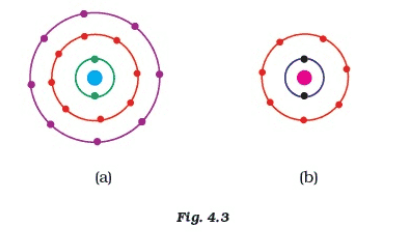Atom (a) has zero valency because it has established a stable configuration with 8 electrons in the valence shell.

The valency of atom (b) is 1 since the valence shell contains 7 electrons. In order to obtain a stable (octet) configuration, atom (b) can take one additional electron.

Q6: One electron is present in the outer most shell of the atom of an element X. What would be the nature and value of charge on the ion formed if this electron is removed from the outer most shell?

When an electron is removed from the outermost shell, a cation is formed, and the element’s charge is increased to +1.

When a single electron from the outermost shell of an element X is removed, the atom loses its negative charge and becomes a positively charged ion with a charge of +1.

The net charge on the ion will be equal to the amount of charge present on one electron.

Q7: Write down the electron distribution of chlorine atom. How many electrons are there in the L shell? (Atomic number of chlorine is 17).

Chlorine has an atomic number of 17. As a result, the innermost shell contains 2 electrons, the second shell has 8 electrons, and the outermost shell has 7 electrons.

K shell – 2 electrons

L shell – 8 electrons

M shell – 7 electrons

The electron configuration of chlorine can be written as 1s22s22p63s23p5 or as [Ne]3s23p5.

Q8: In the atom of an element X, 6 electrons are present in the outermost shell. If it acquires noble gas configuration by accepting requisite number of electrons, then what would be the charge on the ion so formed?

The electronic configuration of a noble gas is 2,8. It possesses 8 electrons in its outermost orbitals. Element X contains 6 electrons in its outermost shell. Two more electrons are required to obtain the noble gas configuration. As a result, in order to achieve a noble gas configuration, the element should take two electrons, resulting in a charge of -2.

Q9: What information do you get from the Fig. 4.4 about the atomic number, mass number and valency of atoms X, Y and Z? Give your answer in a tabular form.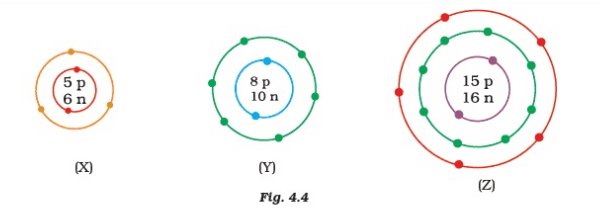Atomic number, mass number and valency of atoms X, Y and Z.

Atomic no Mass no Valency
X 5 11 3
Y 8 18 2
Z 15 31 3.5

Q10: In response to a question, a student stated that in an atom, the number of protons is greater than the number of neutrons, which in turn is greater than the number of electrons. Do you agree with the statement? Justify your answer.

The provided statement is not correct.

According to this comment p > n > e.

The number of protons, on the other hand, is never greater than the number of neutrons.

Because mass number is equal to double the atomic number or greater than double the atomic number, the number of neutrons might be equal to or greater than the number of protons.

Because the number of electrons equals the number of protons in the neutral atom, the number of neutrons can be more than the number of electrons.

Q11: Calculate the number of neutrons present in the nucleus of an element X which is represented as 31X15.

Mass number (A) = No. of protons (Z) + No. of neutrons

Given, the mass number as 31 and the number of protons is 15.

No. of protons (Z) + No. of neutrons = 31

∴ Number of neutrons = 31 – number of protons

⇒ Number of neutrons = 31 – 15 = 16.

Q12: Match the names of the Scientists given in column A with their contributions towards the understanding of the atomic structure as given in column B.

(A) (B)
(a) Ernest Rutherford (i) Indivisibility of atoms
(b) J.J.Thomson (ii) Stationary orbits
(c) Dalton (iii) Concept of the nucleus
(d) Neils Bohr (iv) Discovery of electrons
(e) James Chadwick (v) Atomic number
(f) E. Goldstein (vi) Neutron
(g) Mosley (vii) Canal rays

(a) Ernest Rutherford – (iii) Concept of the nucleus

(b) J.J.Thomson – (iv) Discovery of electrons

(c) Dalton – (i) Indivisibility of atoms

(d) Neils Bohr – (ii) Stationary orbits

(e) James Chadwick – (vi) Neutron

(f) E. Goldstein – (vii) Canal rays

(g) Mosley – (v) Atomic number

Q13: The atomic number of calcium and argon are 20 and 18 respectively, but the mass number of both these elements is 40. What is the name given to such a pair of elements?

They are called isobars.

Isobars have different atomic numbers (or protons), but the same mass number.

Q14: Complete Table 4.1 on the basis of information available in the symbols given below.

(a) 3517Cl

(b) 126C

(c) 8135Br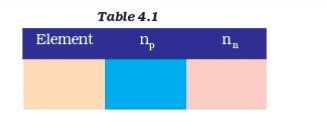Element np nn
Cl 17 18
C 6 6
Br 35 46

Q15: Helium atom has 2 electrons in its valence shell but its valency is not 2, Explain.

Electronic arrangement of a helium atom is 1s2 which contains two valence electrons, but because it does not form a chemical bond, its duplet is complete. As a result, valency is zero.

Q16: Fill in the blanks in the following statements:

(a) Rutherford’s α-particle scattering experiment led to the discovery of the _______.

(b) Isotopes have same ________ but different________.

(c) Neon and chlorine have atomic numbers 10 and 17 respectively. Their valencies will be _______ and ________ respectively.

(d) The electronic configuration of silicon is _______ and that of sulphur is ________.

a) Rutherford’s α-particle scattering experiment led to the discovery of the atomic nucleus.

b) Isotopes have same atomic number but different mass number.

c) Neon and chlorine have atomic numbers 10 and 17 respectively. Their valencies will be 0 and 1 respectively.

d) The electronic configuration of silicon is 2.8.4 and that of sulphur is 2.8.6

Q17: An element X has a mass number 4 and atomic number 2. Write the valency of this element.

Mass number = 4

Atomic number = 2

X is Helium.

It has 0 valency and it would not react with any other atom because it has its outer shell filled.

Q1: Why do Helium, Neon and Argon have a zero valency?

An element’s valency is its ability to combine. The valency of noble gases is zero since there are no free electrons in the valence shell and the elements are already in a stable state.

• The outermost orbit of helium has two electrons, filling shell 1 and generating a duplet arrangement in the valence shell.
• The valence orbit of neon has eight electrons, completing the duplet structure.
• The outermost layer of Argon and Neon has 8 electrons, completing the octet arrangement.

Because these elements have the most electrons in their valence shell, they have a stable electron configuration and do not participate in chemical reactions.

Q2: The ratio of the radii of hydrogen atom and its nucleus is ~ 105. Assuming the atom and the nucleus to be spherical,

(i) What will be the ratio of their sizes?

(ii) If atom is represented by planet earth ‘Re’ = 6.4 ×106m, estimate the size of the nucleus.

Assuming the atom and the nucleus to be spherical,

(i) Atomic size is represented in terms of atomic radius

$$\begin{array}{l}\frac{r_{H}}{r_{n}} = 10^{5}\end{array}$$

Volume of the sphere =

$$\begin{array}{l}\frac{4}{3}\pi r^{3}\end{array}$$
$$\begin{array}{l}V_{H} = \frac{4}{3}\pi r_{H}^{3}\end{array}$$
………………….. (1)

$$\begin{array}{l}V_{n} = \frac{4}{3}\pi r_{n}^{3}\end{array}$$
……………………. (2)

Ratio of volumes

$$\begin{array}{l}=\frac{V_{H}}{V_{n}} = \frac{\frac{4}{3}\pi r_{H}^{3}}{\frac{4}{3}\pi r_{n}^{3}}\end{array}$$
$$\begin{array}{l}=\frac{V_{H}}{V_{n}} = \frac{r_{H}^{3}}{r_{n}^{3}}\end{array}$$
$$\begin{array}{l}=\frac{V_{H}}{V_{n}} = \left ( \frac{r_{H}}{r_{n}} \right )^{3}\end{array}$$
$$\begin{array}{l}=\frac{V_{H}}{V_{n}} = \left ( 10^{5} \right )^{3}\end{array}$$

$$\begin{array}{l}\frac{V_{H}}{V_{n}} = 10^{15}\end{array}$$

(ii) If an atom is represented by planet earth ‘Re’ = 6.4×106m, then the radius of the nucleus would be rn.

$$\begin{array}{l}r_{n} = \frac{r_{e}}{10^{5}}\end{array}$$
$$\begin{array}{l}r_{n} = \frac{(6.4×10^{6})}{10^{5}}\end{array}$$
$$\begin{array}{l}r_{n} = 6.4 x 10\end{array}$$

$$\begin{array}{l}r_{n} = 64m\end{array}$$

Q3: Enlist the conclusions drawn by Rutherford from his α-ray scattering experiment.

The α-particle scattering experiment led Rutherford to the following conclusions:–

(i) Because most α-particles travelled through the gold foil with no deflection, most of the space inside the atom was unoccupied.

(ii) The positive charge occupied very little space inside the atom, as just a few particles were deviated from their route.

(iii) Only a small percentage of α-particles were deflected by 180°, implying that the positive charge and mass of the gold atom are concentrated in a compact volume within the atom.

(iv) He also computed that the radius of the nucleus was approximately 105 times smaller than the radius of the atom based on the data.

Q4: In what way is the Rutherford’s atomic model different from that of Thomson’s atomic model?

Rutherford proposed a model in which the electrons moved in well-defined orbits around the nucleus. An atom has a positively charged centre (later named the “nucleus”). He also proposed that the nucleus is very small in comparison to the size of the atom, and that the nucleus contains nearly all of an atom’s mass.

Thomson recommended that the atom be modelled after a Christmas pudding. The electrons are scattered about like currants in a positively charged pudding spherical, and the atom’s mass was expected to be evenly distributed.

Q5: What were the drawbacks of Rutherford’s model of an atom?

Rutherford carried out an experiment in which he bombarded a thin sheet of gold with -particles and then analysed the trajectory of the particles after they collided with the gold foil.

• Rutherford’s atomic model could not explain how the moving electrons were able to maintain their orbit.
• Any charged particle would give forth energy during acceleration, lose energy while revolving, and eventually fall into the nucleus.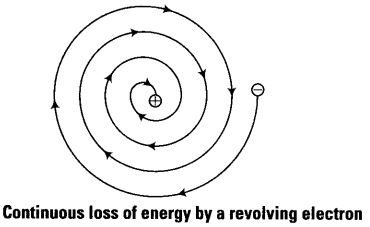Q6: What are the postulates of Bohr’s model of an atom?

The following are the postulates of Bohr’s atom model:

1. Electrons circle the nucleus of an atom in a fixed path known as the orbit or stationary state of the shell.

2. The shells have varying energy levels, represented by the letters K, L, M, and N.

3. The electron does not absorb or release energy as long as it is in an orbit.

4. The electron can only move in orbits where angular momentum is quantized, that is, where the electron’s angular momentum is an integral multiple of h/2𝜋.

Q7: Show diagrammatically the electron distributions in a sodium atom and a sodium ion and also give their atomic number.

The atomic number (Z) of an element is equal to the number of protons in its atom.

Atomic number of sodium (Z) = 11 and Mass number of sodium (A) = 23

Number of protons in the nucleus = 11

Number of neutrons in the nucleus = 23 − 11 = 12

Number of electrons = 11

Thus, electronic configuration of Na-atom = 2, 8, 1(K,L,M)

An electron is lost from the sodium atom(present in the outermost shell), resulting in the formation of the Na+ ion.

Hence, the electronic configuration is 2, 8(K, L). However, the number of protons and neutrons remains the same.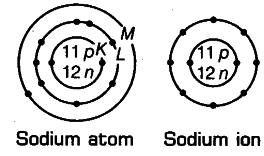Q8: In the Gold foil experiment of Geiger and Marsden, that paved the way for Rutherford’s model of an atom, ~ 1.00% of the α-particles were found to deflect at angles > 50°. If one mole of α-particles were bombarded on the gold foil, compute the number of α-particles that would deflect at angles less than 50°.

% of α-particles deflected more than 50° = 1% of α-particles.

∴ % of α-particles deflected less than 50° = 100 – 1 = 99%

Number of α-particles bombarded = 1 mole = 6.022 × 1023 particles

∴ Number of particles that deflected at an angle less than 50° = 99/100 × 6.022 × 1023

= 5.96 × 1023

### CBSE Class 9 Science Chapter 4 MCQs

1. _______discovered the electron.

(b) Rutherford

(c) Thomson

(d) Goldstein

2. What property of an element determines its chemical behaviour?

(a) Valency of an element

(b) Size of an element

(c) Molar mass of the element

(d) None of the above

Answer: (a) Valency of an element

3. _______ is the radioactive element used in the treatment of cancer.

(a) Iodine-131

(b) Uranium-234

(c) Plutonium-239

(d) Cobalt-60